Question

# A ball is thrown straight upward in the air at a speed of 15.0 m/s. Ignore air resistance.

A ball is thrown straight upward in the air at a speed of 15.0 m/s. Ignore air resistance.

a) What is the maximum height the ball will reach?

b) What is the speed of the ball when it reaches 5.00 m?

c) How long will the ball take to reach 5.00 m above its initial position on the way up?

d) How long will the ball take to reach 5,00 m above its initial position on its way down?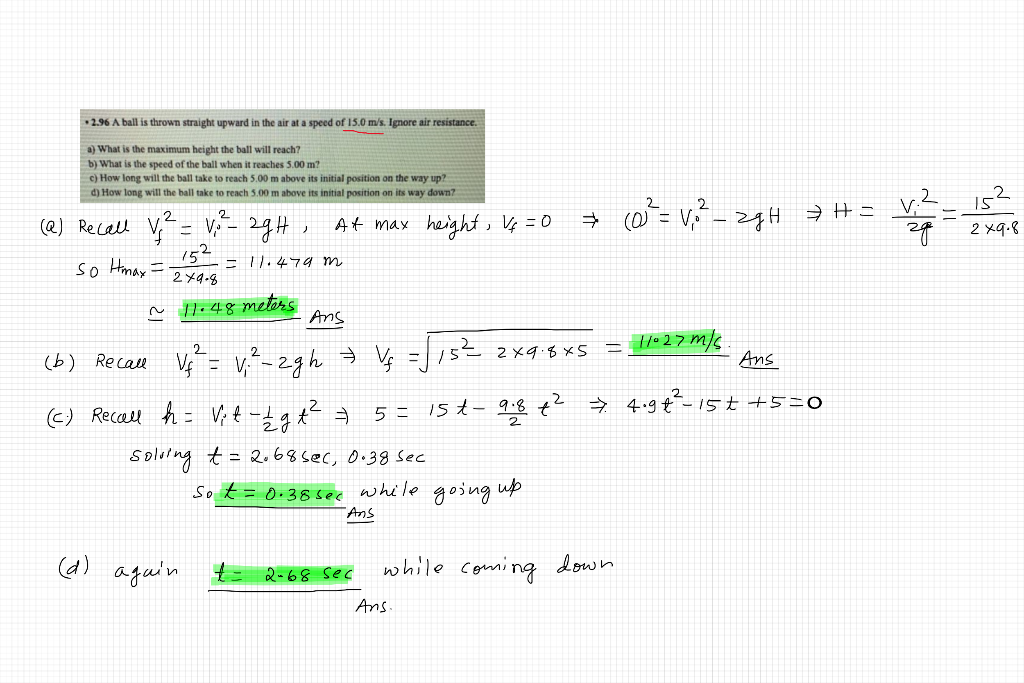#### Earn Coins

Coins can be redeemed for fabulous gifts.

Similar Homework Help Questions
• ### Ba Example 2-16 Ball thrown upward A woman throws a ball upward into the air with...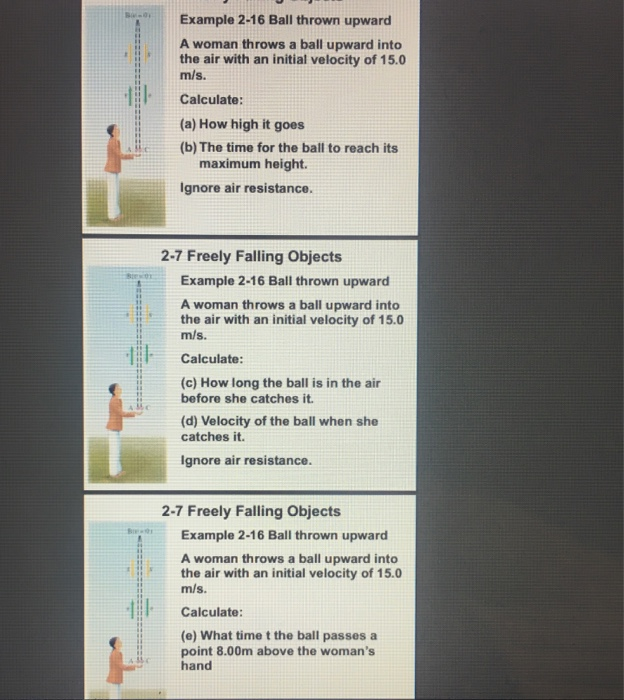Ba Example 2-16 Ball thrown upward A woman throws a ball upward into the air with an initial velocity of 15.0 m/s. Calculate: (a) How high it goes (b) The time for the ball to reach its maximum height Ignore air resistance. 2-7 Freely Falling Objects Example 2-16 Ball thrown upward A woman throws a ball upward into the air with an initial velocity of 15.0 m/s. Calculate: (c) How long the ball is in the air before she catches...

• ### A ball is thrown directly upward and experiences no air resistance. The ball is thrown with...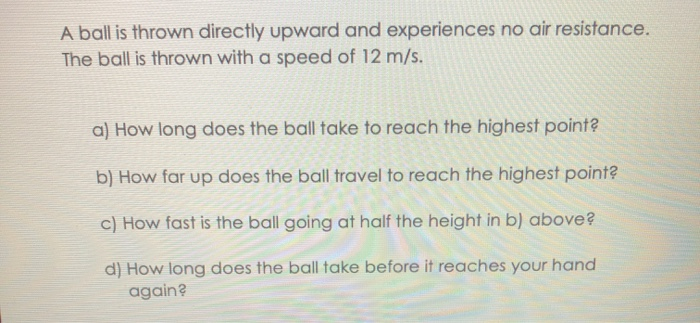A ball is thrown directly upward and experiences no air resistance. The ball is thrown with a speed of 12 m/s. a) How long does the ball take to reach the highest point? b) How far up does the ball travel to reach the highest point? c) How fast is the ball going at half the height in b) above? d) How long does the ball take before it reaches your hand again?

• ### thank you A ball is thrown directly upward and experiences no air resistance. The ball is...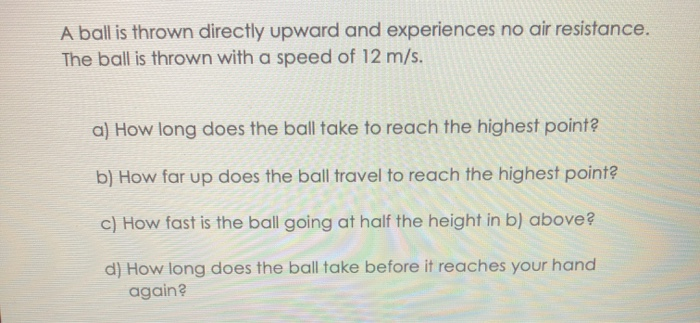thank you A ball is thrown directly upward and experiences no air resistance. The ball is thrown with a speed of 12 m/s. a) How long does the ball take to reach the highest point? b) How far up does the ball travel to reach the highest point? c) How fast is the ball going at half the height in b) above? d) How long does the ball take before it reaches your hand again?

• ### Two throw A blue ball is thrown upward with an initial speed of 22.2 m/s, from...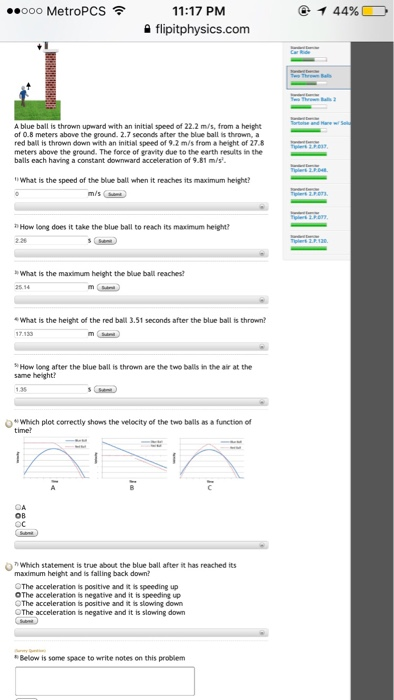Two throw A blue ball is thrown upward with an initial speed of 22.2 m/s, from a height of 0.8 meters above the ground. 2.7 seconds after the blue ball is thrown, a red ball is thrown down with an initial speed of 9.2 m/s from a height of 27.8 meters above the ground. The force of grandly due to the earth results in the balls each having a constant downward acceleration of 9.81 m/s^2. What is the speed of...

• ### A ball is thrown vertically upward with a speed of 15.0 m/s

A ball is thrown vertically upward with a speed of 15.0 m/s. (a) How high does it rise? (b) How long does it take to reach its highest point? (c) How long does the ball take to hit the ground after it reaches its highest point? (d) What is its velocity when it returns to the level from which it started?

• ### A ball is thrown straight up in the air with an initial speed of 40 m/s....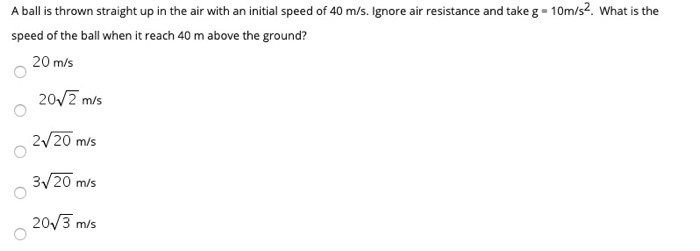A ball is thrown straight up in the air with an initial speed of 40 m/s. Ignore air resistance and take g - 10m/s2. What is the speed of the ball when it reach 40 m above the ground? 20 m/s 2012 m/s 2/20 m/s 3720 m/s 20/3 mis

• ### A red ball is thrown down with an initial speed of 1.2 m/s from a height...

A red ball is thrown down with an initial speed of 1.2 m/s from a height of 25 meters above the ground. Then, 0.6 seconds after the red ball is thrown, a blue ball is thrown upward with an initial speed of 23.8 m/s, from a height of 0.8 meters above the ground. The force of gravity due to the earth results in the balls each having a constant downward acceleration of 9.81 m/s^2. 1)What is the speed of the...

• ### A red ball is thrown down with an initial speed of 1.2 m/s from a height...

A red ball is thrown down with an initial speed of 1.2 m/s from a height of 26 meters above the ground. Then, 0.4 seconds after the red ball is thrown, a blue ball is thrown upward with an initial speed of 23.8 m/s, from a height of 1 meters above the ground. The force of gravity due to the earth results in the balls each having a constant downward acceleration of 9.81 m/s2. 1) What is the speed of...

• ### A ball is thrown vertically upwards with an initial velocity of 18.80 m/s, from the ground....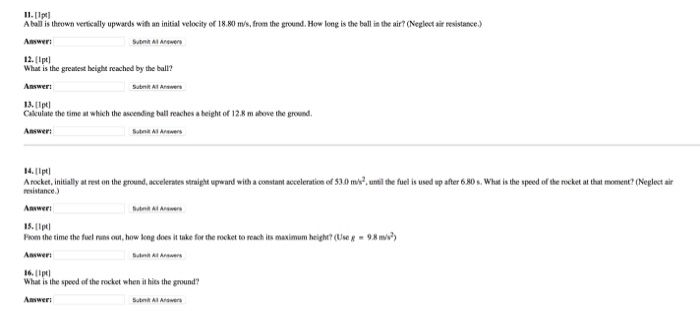A ball is thrown vertically upwards with an initial velocity of 18.80 m/s, from the ground. How long is the ball in the air? (Neglect air resistance.) What is the greatest height reached by the ball? Calculate the time at which the ascending ball reaches a height of 12.8 m above the ground. A rocket, initially at rest on the ground, accelerates straight upward with a constant acceleration of 53.0 m/s^2, until the fuel is used up after 6.80s. What...

• ### A blue ball is thrown upward with an initial speed of 19.8 m/s, from a height of 0.5 meters above the ground

A blue ball is thrown upward with an initial speed of 19.8 m/s, from a height of 0.5 meters above the ground. 2.4 seconds after the blue ball is thrown, a red ball is thrown down with an initial speed of 7.9 m/s from a height of 22.5 meters above the ground. The force of gravity due to the earth results in the balls each having a constant downward acceleration of 9.81 m/s2. What is the maximum height the blue...